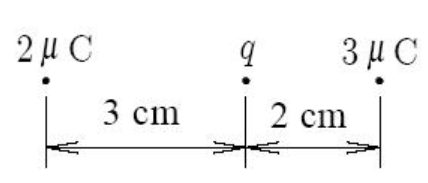# Problem: The figure shows a test charge q between the two positive charges.A. Find the force (in newtons) on the test charge for q = 1 µC. Give a positive answer if the force is to the right and a negative answer if the force is to the left.B. Find the electric field (in newtons/coulomb) at the position of the test charge. Again, supply a positive value if the electric field points to the right and a negative value if it points to the left.

###### FREE Expert Solution

From Coulomb's law:

$\overline{){\mathbf{F}}{\mathbf{=}}\frac{\mathbf{k}{\mathbf{q}}_{\mathbf{1}}{\mathbf{q}}_{\mathbf{2}}}{{\mathbf{r}}^{\mathbf{2}}}}$, where k is Coulomb's constant and r is the distance between charges q1 and q2.

93% (43 ratings)###### Problem Details

The figure shows a test charge q between the two positive charges.A. Find the force (in newtons) on the test charge for q = 1 µC. Give a positive answer if the force is to the right and a negative answer if the force is to the left.

B. Find the electric field (in newtons/coulomb) at the position of the test charge. Again, supply a positive value if the electric field points to the right and a negative value if it points to the left.